Question

# 1) photon dropped Eph1 with frequency vph1 on unexcited hydrogen atom on ground state then the...

1) photon dropped Eph1 with frequency vph1 on unexcited hydrogen atom on ground state then the atom ionized anf an electron comes out with kinetic energy Te , if this electron Unite with another ionic hydrogen then a third hydrogen atom in first excited level on comes out because of this unite with -3.4eV , and a new photon comes out with 466 λ.

calculate the energy of first photon Eph1 and its frequency vph1 .

2) calculate the velocity of electron 1s an atom with charge Z.

what is the value of Z to make the electron velocity equal to speed of light ?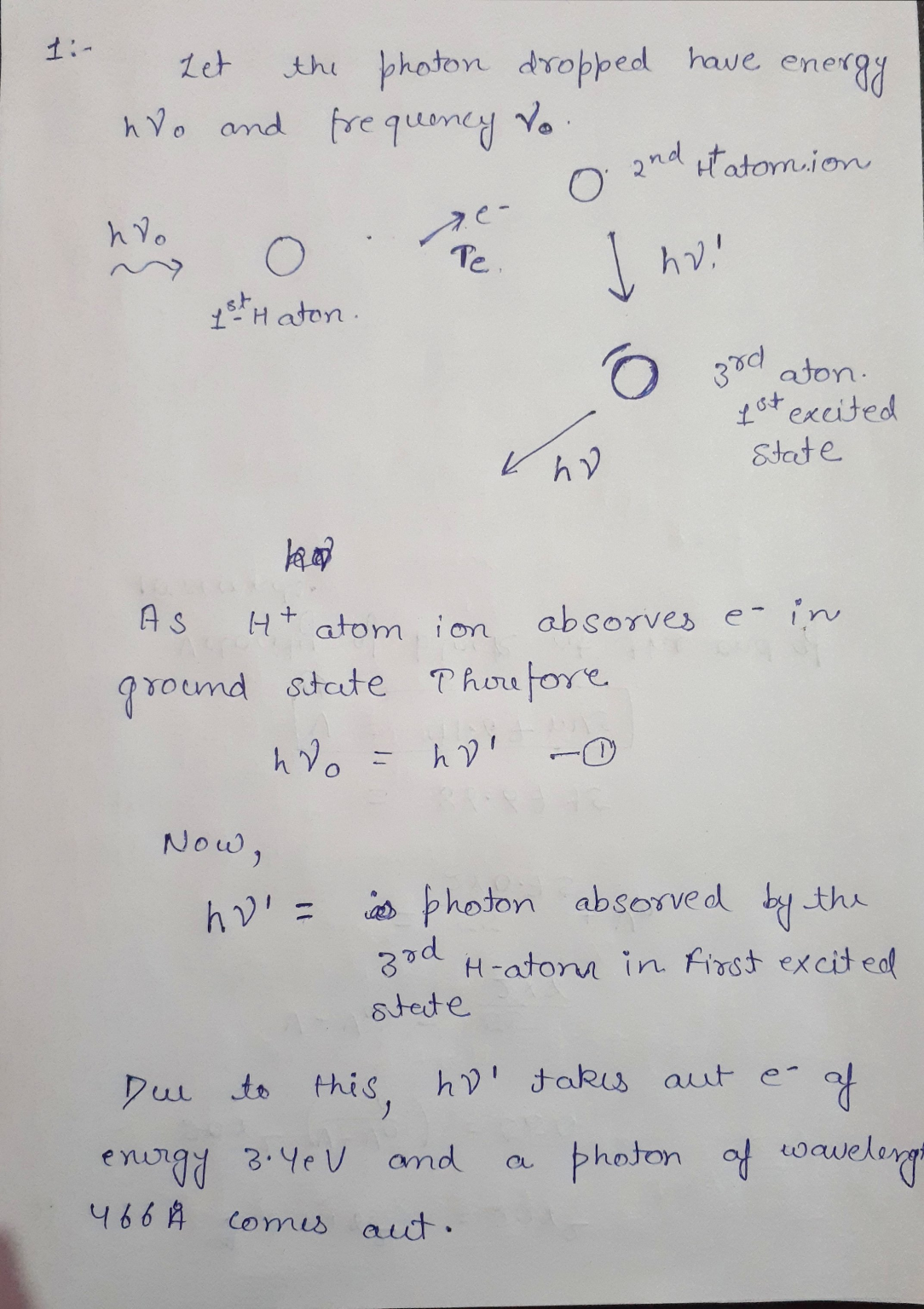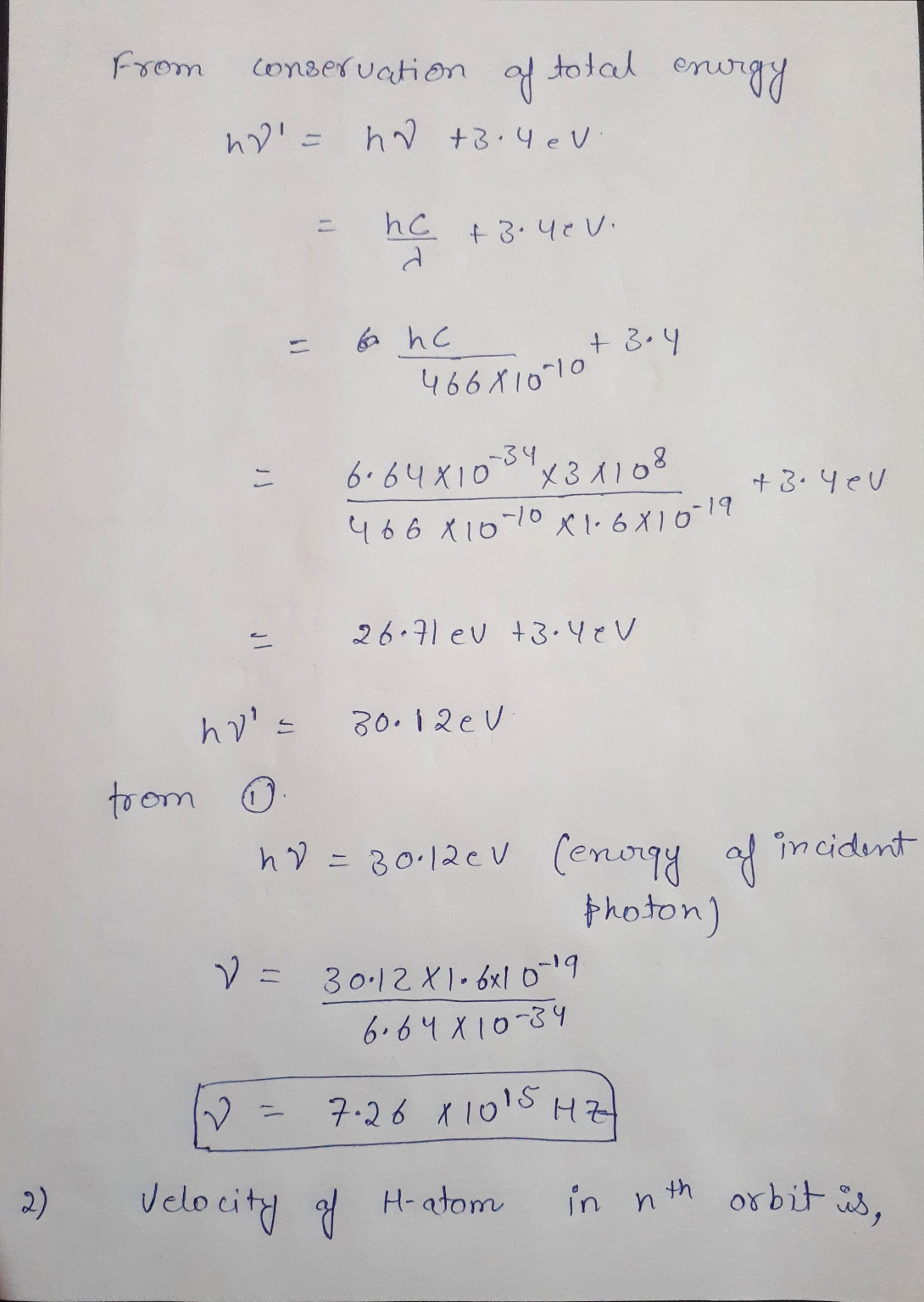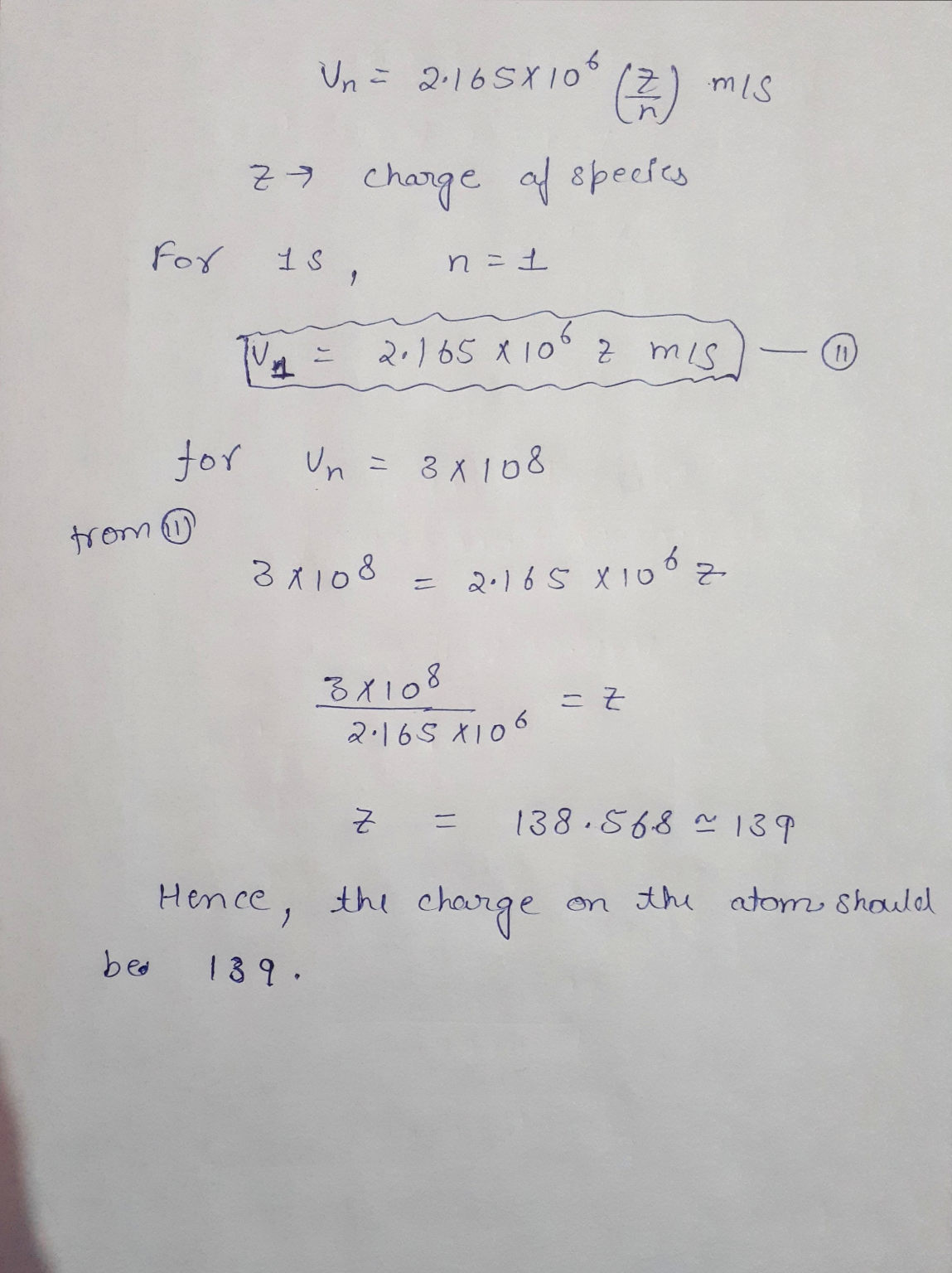#### Earn Coins

Coins can be redeemed for fabulous gifts.

Similar Homework Help Questions
• ### An electron in the ground state of a hydrogen atom (-13.6 eV) absorbs a 10.2 eV...

An electron in the ground state of a hydrogen atom (-13.6 eV) absorbs a 10.2 eV photon and jumps to the first excited state. What is the energy in eV of the first excited state?

• ### Calculate the energy of a photon required to excite a hydrogen atom from the n =...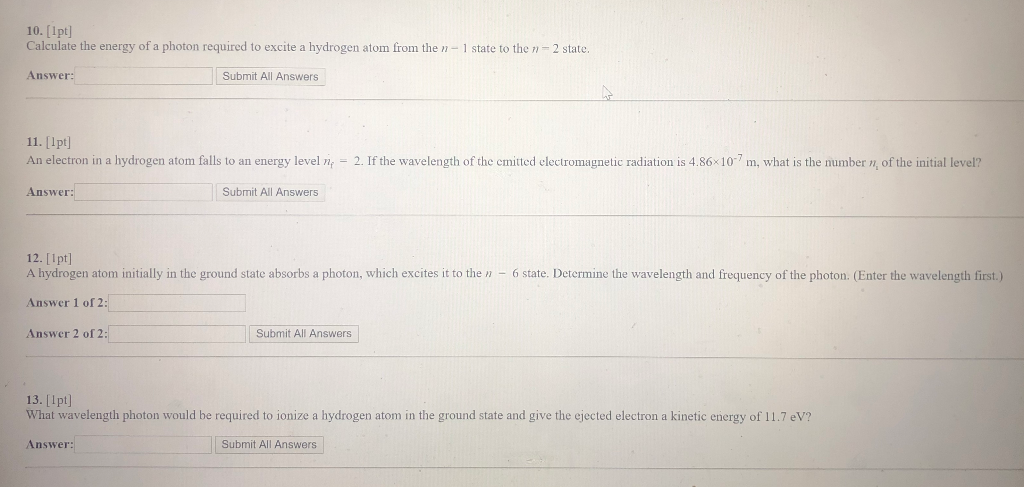Calculate the energy of a photon required to excite a hydrogen atom from the n = 1 state to the n = 2 state. 10. [1pt] Calculate the energy of a photon required to excite a hydrogen atom from the - 1 state to the n - 2 state, Answer: Submit All Answers 11. [1pt] An electron in a hydrogen atom falls to an energy level n = 2. If the wavelength of the emitted electromagnetic radiation is 4.86x10m, what...

• ### A hydrogen atom initially in its ground state i.e., n= 1 level, absorbs a photon and...

A hydrogen atom initially in its ground state i.e., n= 1 level, absorbs a photon and ends up in n= 3 level. What must have been the frequency of the incident photon? (b) Now the electron makes spontaneous emission and comes back to the ground state. What are the possible frequencies of the photons emitted during this process?

• ### A ground state hydrogen atom absorbs a photon of wavelength A. The atom's electron is excited...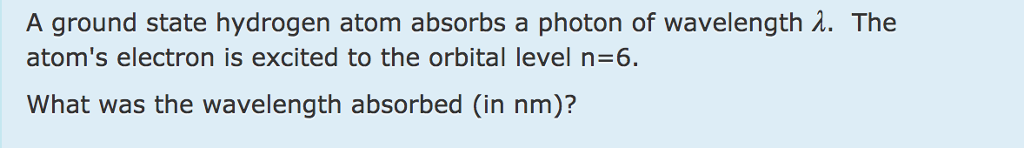A ground state hydrogen atom absorbs a photon of wavelength A. The atom's electron is excited to the orbital level n 6. What was the wavelength absorbed (in nm)?

• ### An electron in the hydrogen atom make a transition from the ground state to an excited...

An electron in the hydrogen atom make a transition from the ground state to an excited level by absorbing energy from a photon. The wavelength of the photon is 95.0 nm. What is the final level that the electron can reach?

• ### Compute the change in energy of the 2p→ 1s photon when a hydrogen atom is placed...

Compute the change in energy of the 2p→ 1s photon when a hydrogen atom is placed in a magnetic field of 2.00 T. 2 III. (12pts) The electron of a hydrogen atom is excited to the n= 5 state. (a) what is the Bohr radius of the electron? (b) what is the total energy of the electron? (c) what is the electron’s Coulomb potential energy and kinetic energy? IV. (12pts) X-ray photons of wavelength 0.120 nm are incident on a...

• ### e) A hydrogen atom is in its ground state (n = 1). Using the Bohr theory...

e) A hydrogen atom is in its ground state (n = 1). Using the Bohr theory of the atom, calculate (e) the energy gained by moving to a state where n = 5. g) A hydrogen atom is in its ground state (n = 1). Using the Bohr theory of the atom, calculate (g) the wavelength, λ, of the EM waved adsorbed in the process of moving the electron to a state where n = 5. Hint: There are two...

• ### A hydrogen atom initially in its ground state absorbs a photon and ends up in the...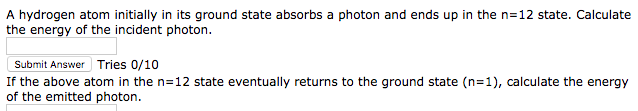A hydrogen atom initially in its ground state absorbs a photon and ends up in the n=12 state. Calculate the energy of the incident photon Submit Answer Tries 0/10 If the above atom in the n=12 state eventually returns to the ground state (n=1), calculate the energy of the emitted photon.

• ### For the hydrogen atom, its energy at ground state is 13.6 eV, at first excited state...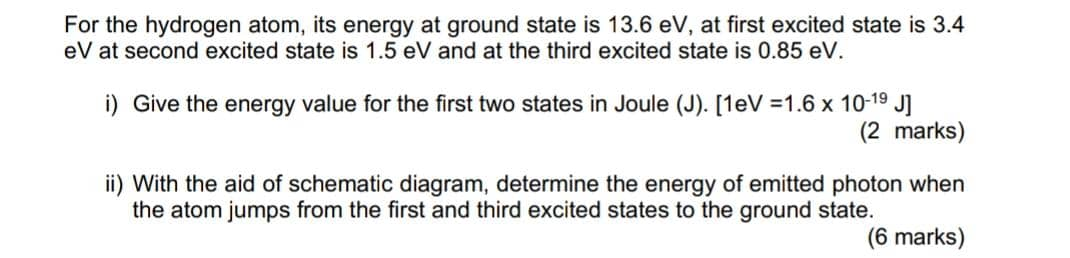For the hydrogen atom, its energy at ground state is 13.6 eV, at first excited state is 3.4 eV at second excited state is 1.5 eV and at the third excited state is 0.85 eV. i) Give the energy value for the first two states in Joule (J). [1eV =1.6 x 10-19 J] (2 marks) ii) With the aid of schematic diagram, determine the energy of emitted photon when the atom jumps from the first and third excited states to...

• ### A photon of wavelength 300 Å is incident upon a hydrogen atom at rest. The photon...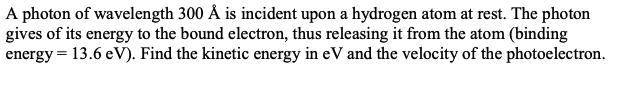A photon of wavelength 300 Å is incident upon a hydrogen atom at rest. The photon gives of its energy to the bound electron, thus releasing it from the atom (binding energy = 13.6 eV). Find the kinetic energy in eV and the velocity of the photoelectron.